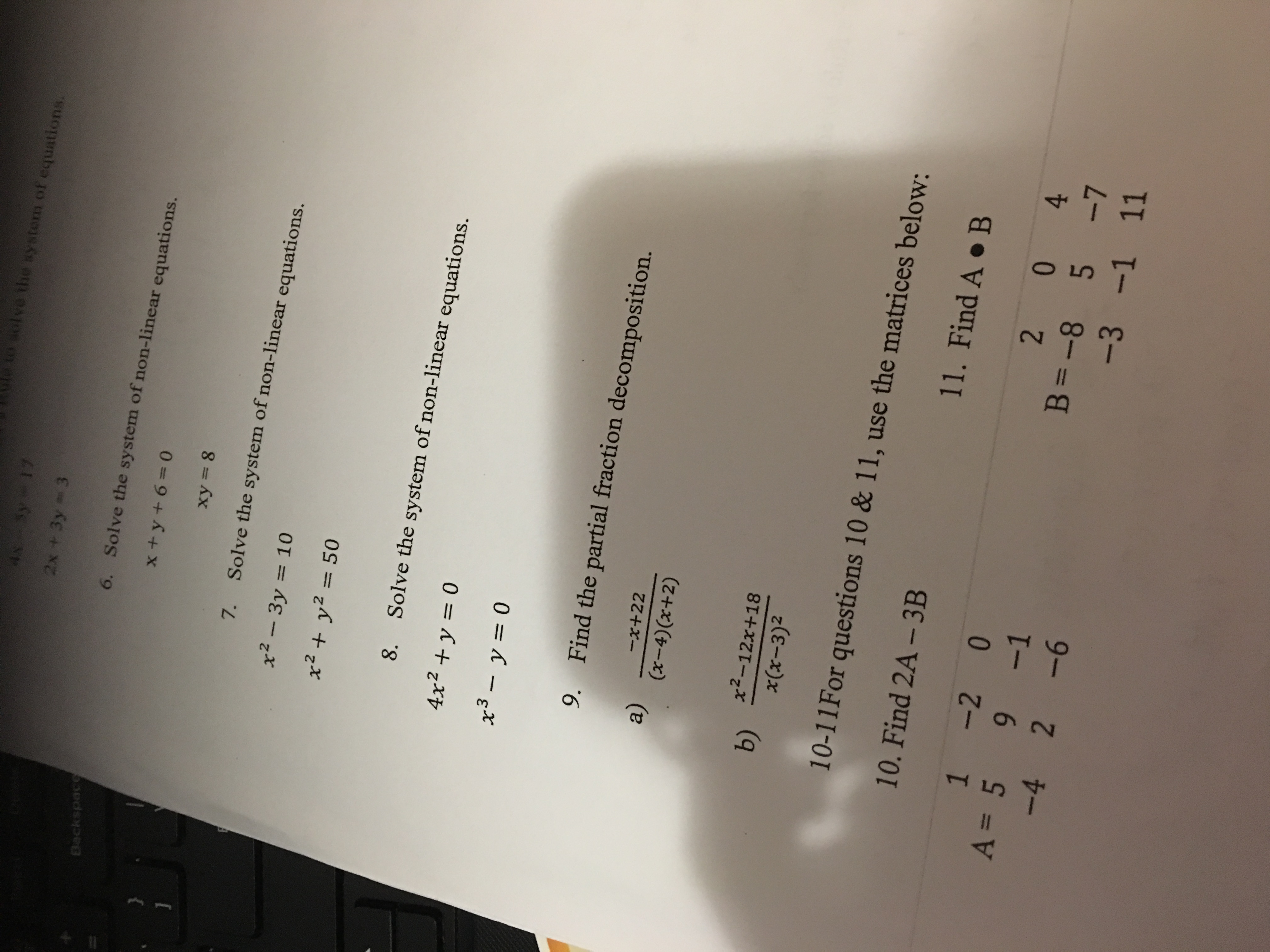# equatio2x +3y 36.Solve the system of non-linear equations.xy- 87. Solve the system of non-linear equations.x2-3y = 10x2+ y2508.Solve the system of non-linear equations.4x2 + y = 09. Find the partial fraction decomposition.a)-x+22(x-4)(r+2)b) 2-12x+1810-11 For questions 10 & 11, use the matrices below:10. Find 2A-3B1 -2 0x(x-3)211 . Find A . ВA=5 9-1-4 2-61B -8 5 71

Question

I need help w/ 9, 10, & 11help_outlineImage Transcriptioncloseequatio 2x +3y 3 6. Solve the system of non-linear equations. xy- 8 7. Solve the system of non-linear equations. x2-3y = 10 x2+ y250 8. Solve the system of non-linear equations. 4x2 + y = 0 9. Find the partial fraction decomposition. a) -x+22 (x-4)(r+2) b) 2-12x+18 10-11 For questions 10 & 11, use the matrices below: 10. Find 2A-3B 1 -2 0 x(x-3)2 11 . Find A . В A=5 9-1 -4 2-6 1 B -8 5 7 1 fullscreen
check_circle

Step 1

We’ll answer the first question i.e. Q.9. Please submit a new question for the remaining.

Step 2

a.

Given fraction is:

The denominator is in factored form.

So the fraction is written using variables as:

Multiplying by (x-4)(x+2) on both sides:

Grouping x terms and constant terms:

Two polynomials are equal when the respective coefficients are equal. So, equating the coefficients:

Solving the system of equations for A and B:

Hence the partial decomposition of the fraction is given by:

Step 3

b.

Given fraction is:

The denominator is in factored form.

So the fraction is written using variables as:

Multiplying by x(x-3)2 on both sides:

This can be solved by plugging different values for x.

Plugging x=0,

Plugging ...

### Want to see the full answer?

See Solution

#### Want to see this answer and more?

Solutions are written by subject experts who are available 24/7. Questions are typically answered within 1 hour.*

See Solution
*Response times may vary by subject and question.
Tagged in

### Algebra